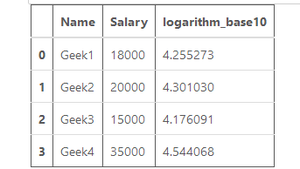Open In App

# Log and natural Logarithmic value of a column in Pandas – Python

Log and natural logarithmic value of a column in pandas can be calculated using the log(), log2(), and log10() numpy functions respectively. Before applying the functions, we need to create a dataframe.

Code:

## Python3

 `# Import required libraries``import` `pandas as pd``import` `numpy as np``  ` `# Dictionary``data ``=` `{``     ``'Name'``: [``'Geek1'``, ``'Geek2'``,``             ``'Geek3'``, ``'Geek4'``],``   ``'Salary'``: [``18000``, ``20000``, ``             ``15000``, ``35000``]} `` ` `# Create a dataframe``data ``=` `pd.DataFrame(data,``                    ``columns ``=` `[``'Name'``, ``                               ``'Salary'``])`` ` `# Show the dataframe``data`

Output:## Logarithm on base 2 value of a column in pandas:

After the dataframe is created, we can apply numpy.log2() function to the columns. In this case, we will be finding the logarithm values of the column salary. The computed values are stored in the new column “logarithm_base2”.

Code:

## Python3

 `# Calculate logarithm to base 2 ``# on 'Salary' column``data[``'logarithm_base2'``] ``=` `np.log2(data[``'Salary'``])`` ` `# Show the dataframe``data    `

Output :## Logarithm on base 10 value of a column in pandas:

To find the logarithm on base 10 values we can apply numpy.log10() function to the columns. In this case, we will be finding the logarithm values of the column salary. The computed values are stored in the new column “logarithm_base10”.

Code:

## Python3

 `# Calculate logarithm to ``# base 10 on 'Salary' column``data[``'logarithm_base10'``] ``=` `np.log10(data[``'Salary'``])`` ` `# Show the dataframe``data`

Output :## Natural logarithmic value of a column in pandas:

To find the natural logarithmic values we can apply numpy.log() function to the columns. In this case, we will be finding the natural logarithm values of the column salary. The computed values are stored in the new column “natural_log”.

Code:

## Python3

 `# Calculate natural logarithm on``# 'Salary' column``data[``'natural_log'``] ``=` `np.log(data[``'Salary'``])`` ` `# Show the dataframe``data    `

Output :